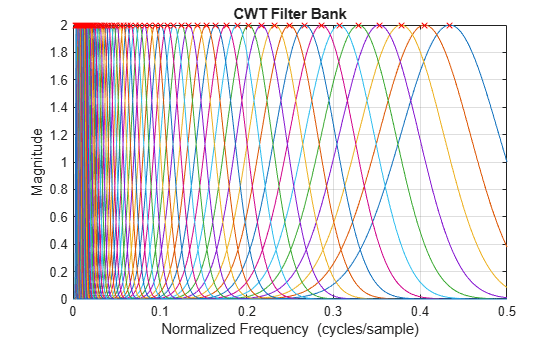# centerFrequencies

CWT filter bank bandpass center frequencies

## Syntax

``bpcf = centerFrequencies(fb)``

## Description

example

````bpcf = centerFrequencies(fb)` returns the wavelet bandpass center frequencies `bpcf` for the CWT filter bank `fb`. Frequencies are ordered from high to low. Frequencies are in cycles/sample if a sampling frequency or sampling period is not specified. If a sampling frequency is specified, `bpcf` has units of hertz. If a sampling period is specified, `bpcf` has units of cycles/unit time, where the time unit is the same as the duration `SamplingPeriod`.```

## Examples

collapse all

Create a CWT filter bank.

`fb = cwtfilterbank;`

Calculate the bandpass center frequencies.

`bpcf = centerFrequencies(fb);`

Plot the frequency responses of the filter bank and the bandpass center frequencies. The bandpass center frequencies correspond to the peaks of the frequency response of each wavelet in the filter bank.

```freqz(fb) hold on plot(bpcf,2*ones(size(bpcf)),'rx')```## Input Arguments

collapse all

Continuous wavelet transform (CWT) filter bank, specified as a `cwtfilterbank` object.

## Output Arguments

collapse all

Wavelet bandpass center frequencies, returned as a real-valued vector of length Ns, where Ns is the number of scales in the filter bank. Frequencies are ordered from high to low. Frequencies are in cycles/sample if a sampling frequency or sampling period is not specified. If a sampling frequency is specified, `bpcf` has units of hertz. If a sampling period is specified, `bpcf` has units of cycles/unit time, where the time unit is the same as the duration `SamplingPeriod`.

## Extended Capabilities

### C/C++ Code GenerationGenerate C and C++ code using MATLAB® Coder™.